Electronic Structure of Atom Homework HelpAre you a university or school looking for an online tutoring partnership ?

Talk to Us

# Instant Homework Help with Electronic Structure of Atoms problems

With the help of our solvers you will be able to relate electron configurations to element classifications in the periodic table and finish your assignments on time.

## Basic concepts in Electronic Structure Of Atom:

### Atomic structure-

Atom is the smallest unit of any substance. It is composed of certain subatomic particles; electrons, neutrons and protons. The arrangement of these subatomic particles in an atom is called the electronic structure of an atom.

### Electronic orbital-

The area around the nucleus with the highest probability of finding an electron is known as the electronic orbital. The Atomic physics and Quantum mechanics support the presence of electronic orbitals.

### Electronic configuration-

This is the distribution of electrons in various electronic orbitals around the nucleus. Electrons always occupy lower energy levels then move to higher energy levels.

### Rules for electronic configuration-

The distribution of electrons in various orbitals follow certain rules as mentioned below:

• Hund’s rule: Every electron in a subshell will be single until the empty orbitals are available in the same subshell.
• Aufbau principle: Electrons always occupy the lower energy level and then move to higher energy levels.
• Pauli’s exclusion principle: No two electrons in an atom can have the same quantum numbers.

### Quantum number-

Four quantum numbers are used to explain the position and energy of electrons in an atom.

• Principle quantum number: Represents as “n” and used to calculate the energy and shell number of electrons.
• Azimuthal quantum number: Denoted by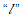and is used to calculate the position of an electron in a certain subshell.
• Magnetic quantum number: Denoted by “m” and represents the magnetic moment of an electron.
• Spin quantum number: Related to the spin of an electron and denoted by ‘s’. The value of ‘s’ is always +½ or -½ .

### Electron orbital energy-

Bohr’s atomic model explained the total energy of electrons as the sum of their potential energy and kinetic energy. The mathematical expression of orbital energy is: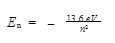### Rydberg equation-

Rydberg equation is used to calculate the wavelength and energy of electrons in transition from initial energy level to final energy level. The mathematical expression is: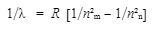Here: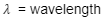R = Rydberg constant = 1.097 x 10⁷ m⁻¹
nₘ = Initial energy level
nₙ= Final energy level

Radius of atomic orbitals can be calculated with the help of value of “n” quantum number.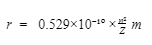Here; r = radius, n = principle quantum number, Z = atomic number

## Sample Questions and Answers on Electronic Structure of Atoms:

Question 1: Identify the correct energy order for the electronic structure of an atom.

• 2s < 2p< 3s< 3p< 3d< 3f
• 1s< 2s< 2p< 3p
• 1s< 2s< 3s< 3p<3d
• 1s<2s<2p<3s<3p<4s<3d

Question 2: Calculate the radius of the second atomic orbital of He atom.

Solution:n = principle quantum number  2
Z = atomic number of He = 2
Substitute the values to calculate radius:r =1.058 ×10⁻¹º m

Question 3: Calculate the wavelength in nanometers, if the electron in the hydrogen atom moves from n=2 to n=3.

Solution: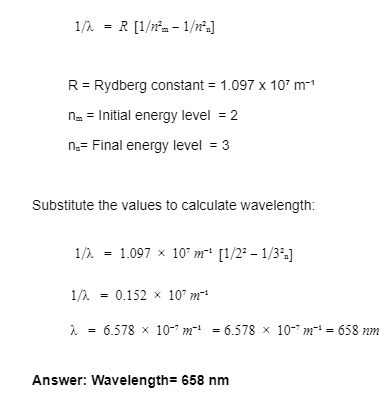## Get reliable answers for electronic structures, distribution and configuration problems:

Atoms are the basic units of matter and the smallest units in the universe that cannot be divided. Here at TutorEye, we’ll help you decode even subatomic particles like nucleons and quarks. Let’s find out all about the building blocks that are in existence since the Big Bang.

Be it Dalton’s atomic theory or Bohr's model of hydrogen - we have a top aide to assist you attempt each question of your school and college assignment.

Right from figuring out electronic structure of carbon, oxygen, helium and aluminum to understanding valence electrons to ionic compounds- you have a reliable chemistry expert to depend upon.

Our Homework assistance service is designed to help you piece together different atomic structures.Whether you are in high school or college- it’s great to have a reference material with diagrams to consult upon when you need to remember the so-called difficult stuff!.

If you are a homeschooler or an active parent supervising your child’s study needs- we’ll help you follow the postulates of various theories by citing enough examples.

As we make efforts to explain chemical and physical properties of a substance in terms of the structure and bonding of molecules- we also provide illustrated examples that can be used as reference materials for tests and examinations.

In addition to this, get textmaps with a set of instructions to solve question banks and old question papers for exams. By taking timely help, you can put rest to your worries and enjoy learning.

## Follow the few simple steps to gain comprehensive understanding of atomic structure:

To understand orbital electronic structures, you need our homework solvers to demonstrate the problems by providing you stepwise answers. Whether it is help with labelling parts of atoms diagram, or assistance with locating elements on periodic table- we provide 100% original work.

Follow these few simple steps to get Chemistry help:

Step 1: Upload your electronic structure of atom related problems and fill out details on our quick resolution form

Step 2: Out of the many proposals, hire an expert who quotes a reasonable price and agrees to the turnaround time

Step 3: Check your assignment carefully before releasing payment from escrow

At TutorEye, we believe in providing affordable and quality online tutoring to our student community. Our work is plagiarism-free and we take steps to ensure multiple revisions at our end before we submit the final draft. We have a Money Refund policy as we value your trust and satisfaction at all costs.

## We Are Different from Others!